Multiply and Divide Whole Numbers

•WatchEverything You Need to Know About Long Multiplication
•WatchEverything You Need to Know about Long Division
•WatchThe Steps to Long Division
•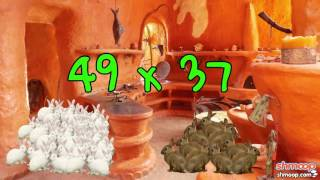WatchMath Basics: Long Multiplication
•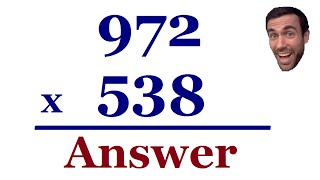WatchMultiplying - Large numbers
•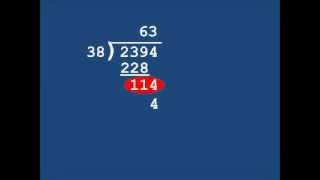WatchLong Division With Two Digit Divisor
•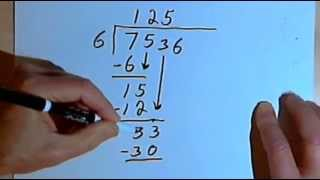WatchLong Division - dividing by a 1-digit number 127-2.10
•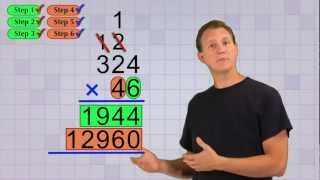WatchMath Antics - Multi-Digit Multiplication Pt 2
•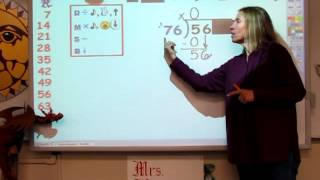WatchDividing by a 2-digit divisor
•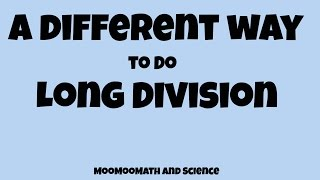WatchA different way to do long division-Math made easy
•WatchBox Method for Long Division!
•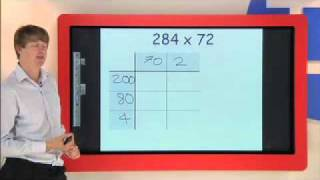WatchLong Multiplication using the Grid (or Box) Method
•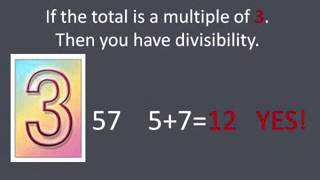WatchRules for divisibility NEW
•WatchDivisibility Song
•WatchSpecial K - Divisibilty Rules
•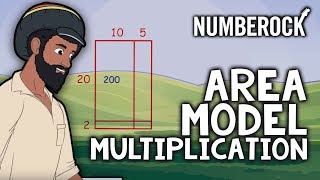WatchArea Model Multiplication Song | 3rd - 4th Grade
•WatchMultiplication Mash Up - A Fun Way to Learn Your Multiplication Facts!
•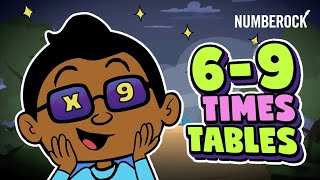WatchTimes Tables Songs For Kids | 6, 7, 8 & 9 | Fun Multiplication Songs
•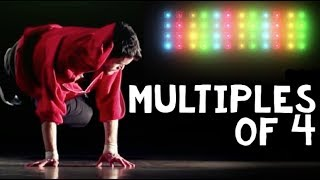WatchSkip Counting by 4 Rap Song | Dance the 4 Times Tables
•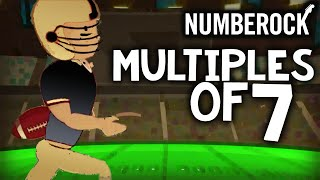Watch7 Times Table Song Rap: Skip Counting by 7 | Multiplication Song by NUMBEROCK
•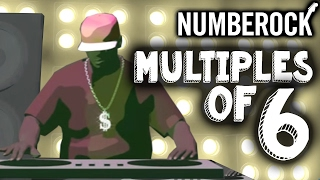Watch6 Times Table Song | Skip Counting by 6 | Multiplication Song
•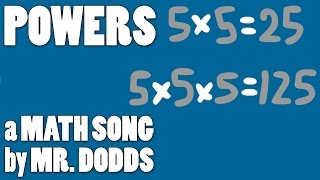WatchColin Dodds - Powers (Math Song)
•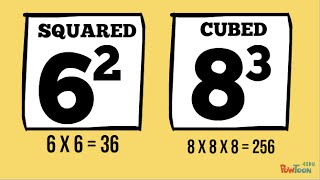WatchExponents Song (All About the Base)
•WatchPrivate video
•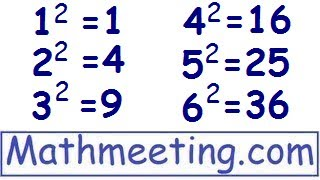WatchExponents - Introduction
•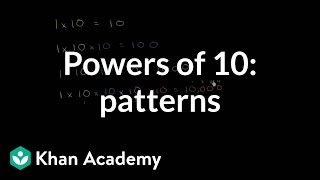WatchPowers of 10: patterns | Place value and decimals | 5th grade | Khan Academy
•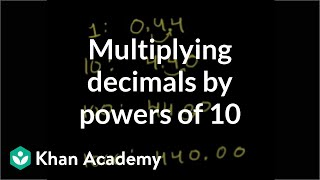WatchMultiplying a decimal by a power of 10 | Decimals | Pre-Algebra | Khan Academy
•WatchMultiplying Decimals with Powers of 10 (4.1)
•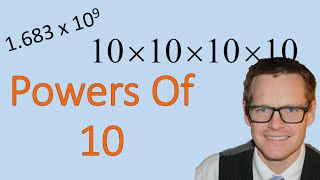WatchPowers of 10 (Simplifying Math)
•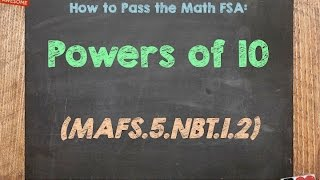WatchHow to Pass the Math FSA (5th) - Powers of 10
•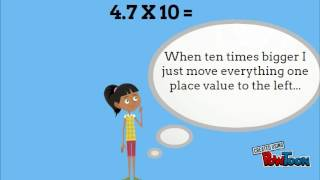Watch5.NBT.1 x10 and 1/10 of
•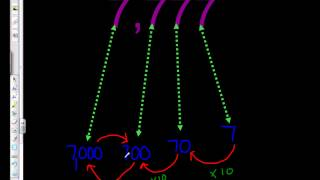WatchVideo Walkthrough: Place Value x 10 to the left x 1/10 to the right 5.NBT.1
•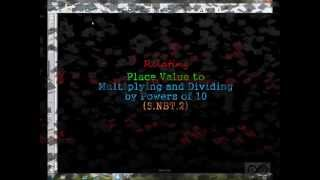WatchRelating Place Value to Multiplying and Dividing by Powers of 10 (5-NBT-2)
•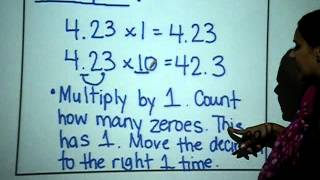WatchPowers of 10 Review and Multiplying Decimals by 10, 100, 1000
•WatchDecimal Shuffle
•WatchDecimal Rap
•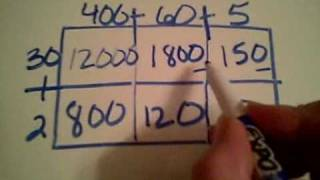WatchBox Multiplication
•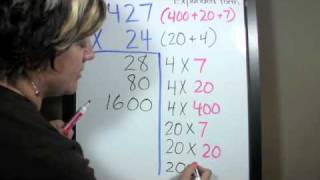WatchPartial Products Multiplication Strategy
•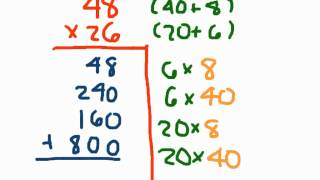WatchPartial Product Multiplication
•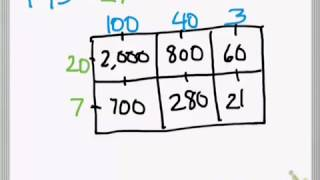WatchMultiplication Area Model Method 3 digit by 2 digit
•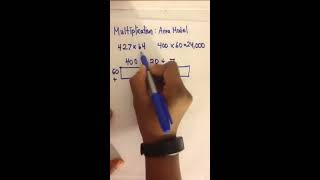WatchMultiplication using an Area Model: 3-Digit x 2-Digit
•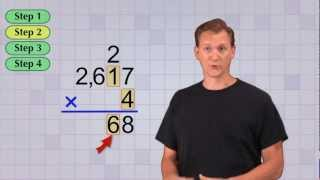WatchMath Antics - Multi-Digit Multiplication Pt 1
•WatchMultiplying Decimals (Models)
•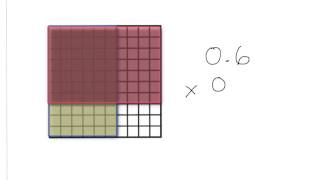Watchlesson 4.6 decimal multiplication models
•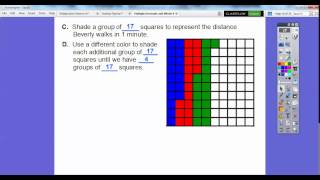WatchMultiply Decimals and Whole Numbers - Lesson 4.2
•Watch5th Go Math! Unit 1, Lesson 4.6
•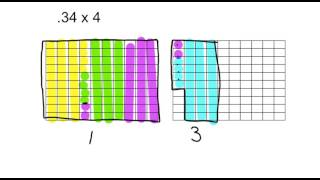Watchmultiplying a decimal by a whole number using base 10 grid
•WatchMultiplying Decimals
•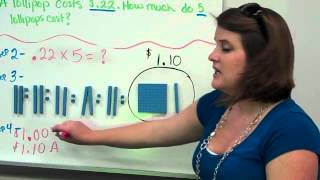WatchMultiplying Decimals Flip Video
•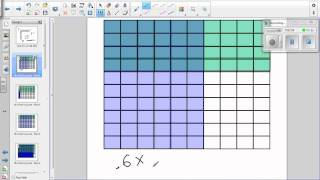WatchModel for Multiplying Decimals Video

Multiplication and Division of Whole Numbers. Multiplication of whole numbers. Multiplication is the repeated addition of the same number. The numbers that are multiplied are called factors. The answer is called the product. The times sign “x” is one symbol that is used to mean multiplication. The basic fact of multiplying …